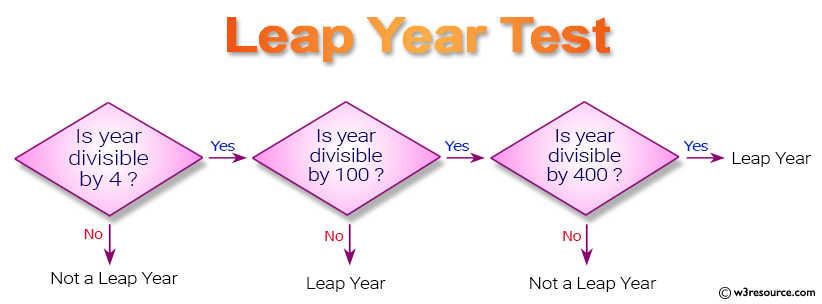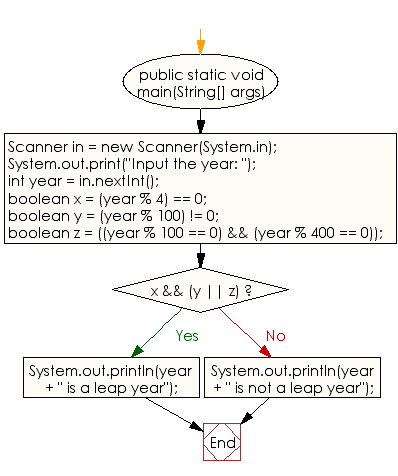﻿ Java - Test a year is a leap year or not

# Java: Test a year is a leap year or not

## Java Conditional Statement: Exercise-9 with Solution

Write a Java program that takes a year from the user and prints whether it is a leap year or not.

Test Data
Input the year: 2016

Pictorial Presentation:Sample Solution:

Java Code:

``````import java.util.Scanner;
public class Exercise9 {

public static void main(String[] args)
{
Scanner in = new Scanner(System.in);

System.out.print("Input the year: ");
int year = in.nextInt();

boolean x = (year % 4) == 0;
boolean y = (year % 100) != 0;
boolean z = ((year % 100 == 0) && (year % 400 == 0));

if (x && (y || z))
{
System.out.println(year + " is a leap year");
}
else
{
System.out.println(year + " is not a leap year");
}
}
}
```
```

Sample Output:

```Input the year: 2016
2016 is a leap year
```

Flowchart:Java Code Editor:

What is the difficulty level of this exercise?

Test your Programming skills with w3resource's quiz.

﻿

## Java: Tips of the Day

IsPowerOfTwo

Checks if a value is positive power of two.

To understand how it works let's assume we made a call IsPowerOfTwo(4).

As value is greater than 0, so right side of the && operator will be evaluated.

The result of (~value + 1) is equal to value itself. ~100 + 001 => 011 + 001 => 100. This is equal to value.

The result of (value & value) is value. 100 & 100 => 100.

This will value the expression to true as value is equal to value.

```public static boolean isPowerOfTwo(final int value) {
return value > 0 && ((value & (~value + 1)) == value);
}
```

Ref: https://bit.ly/3sA5d4I

We are closing our Disqus commenting system for some maintenanace issues. You may write to us at reach[at]yahoo[dot]com or visit us at Facebook